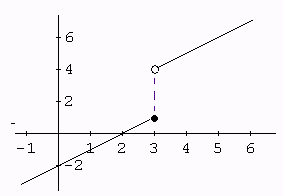# Discontinuity

In Maths, a function f(x) is said to be discontinuous at a point ‘a’ of its domain D if it is not continuous there. The point ‘a’ is then called a point of discontinuity of the function. In limits and continuity, you must have learned a continuous function can be traced without lifting the pen on the graph. The discontinuity may arise due to any of the following situation:

1. The right-hand limit or the left-hand limit or both of a function may not exist.
2. The right-hand limit and the left-hand limit of function may exist but are unequal.
3. The right-hand limit, as well as the left-hand limit of a function, may exist, but either of the two or both may not be equal to f(a).

## Discontinuity in Maths Definition

The function of the graph which is not connected with each other is known as a discontinuous function. A function f(x) is said to have a discontinuity of the first kind at x = a, if the left-hand limit of f(x) and right-hand limit of f(x) both exist but are not equal. f(x) is said to have a discontinuity of the first kind from the left at x = a, if the left hand of the function exists but not equal to f(a).In the above graph, the limits of the function to the left and to the right are not equal and hence the limit at x = 3 does not exist anymore. Such function is said to be a discontinuity of a function.

## Types of Discontinuity

There are three types of discontinuity.

• Jump Discontinuity
• Infinite Discontinuity
• Removable Discontinuity

Now let us discuss all its types one by one.

### Jump Discontinuity

Jump discontinuity is of two types:

• Discontinuity of the First Kind
• Discontinuity of the Second Kind

Discontinuity of the First Kind: Function f(x) is said to have a discontinuity of the first kind from the right at x = a, if the right hand of the function exists but is not equal to f(a). In Jump Discontinuity, the Left-Hand Limit and the Right-Hand Limit exist and are finite but not equal to each other.

Discontinuity of the Second Kind: A function f(x) is said to have discontinuity of the second kind at x = a, if neither left-hand limit of f(x) at x = a nor right-hand limit of f(x) at x = a exists.

### Removable Discontinuity

A function f(x) is said to have a removable discontinuity at x = a, if the left-hand limit at x tends to point ‘a’ is equal to the right-hand limit at x tends to point ‘a’ but their common value is not equal to f(a). A removable discontinuity occurs when there is a rational expression with common factors in the numerator and denominator. Since these factors can be cancelled, the discontinuity is removed.

### Infinite Discontinuity

In Infinite Discontinuity, either one or both Right Hand and Left Hand Limit do not exist or are Infinite. It is also known as Essential Discontinuity. Whenever the graph of a function f(x) has the line x = k, as a vertical asymptote, then f(x) becomes positively or negatively infinite as x→k+ or x→k. Then, function f(x) is said to have an infinite discontinuity.